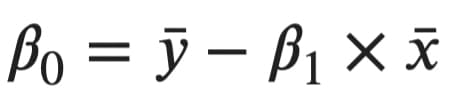# INIT1-ExploreML

Exploring simple linear regression using Python3.

## What it is

It’s an algorithm used by many in introductory machine learning, but it doesn’t require any “learning”. It’s as simple as plugging few values into a formula. In general, linear regression is used to predict continuous variables - something such as stock price, weight, and similar. Linear regression is a linear algorithm, meaning the linear relationship between input variables (what goes in) and the output variable (the prediction) is assumed.

The algorithm is also rather strict on the requirements.

• Linear Assumption - model assumes the relationship between variables is linear
• No Noise - model assumes that the input and output variables are not noisy - so remove outliers if possible
• No Collinearity - model will overfit when you have highly correlated input variables
• Normal Distribution - the model will make more reliable predictions if your input and output variables are normally distributed.
• Rescaled Inputs - use scalers or normalizer to make more reliable predictions

## Explanation

We need to solve the linear equation of the form y = B0 + B1x. Where B0 is the constant and B1 is the slope. The slope can be found using the formula:The Xi represents the current value of the input feature, and X with a bar on top represents the mean of the entire variable. The same goes with Y, but we’re looking at the target variable instead.

And then the constant can be found using:## Setup

• Install Python3 from here.
• Install the required libraries: `python3 -m pip install numpy scipy sklearn`
• Clone the repo: `git clone https://github.com/adviksinghania/init1-exploreml.git`
• Navigate inside the directory: `cd init1-exploreml`
• Run `python3 main.py`

NOTE: This repository/project was made by following the article on Simple Linear Regression by Dario Radečić